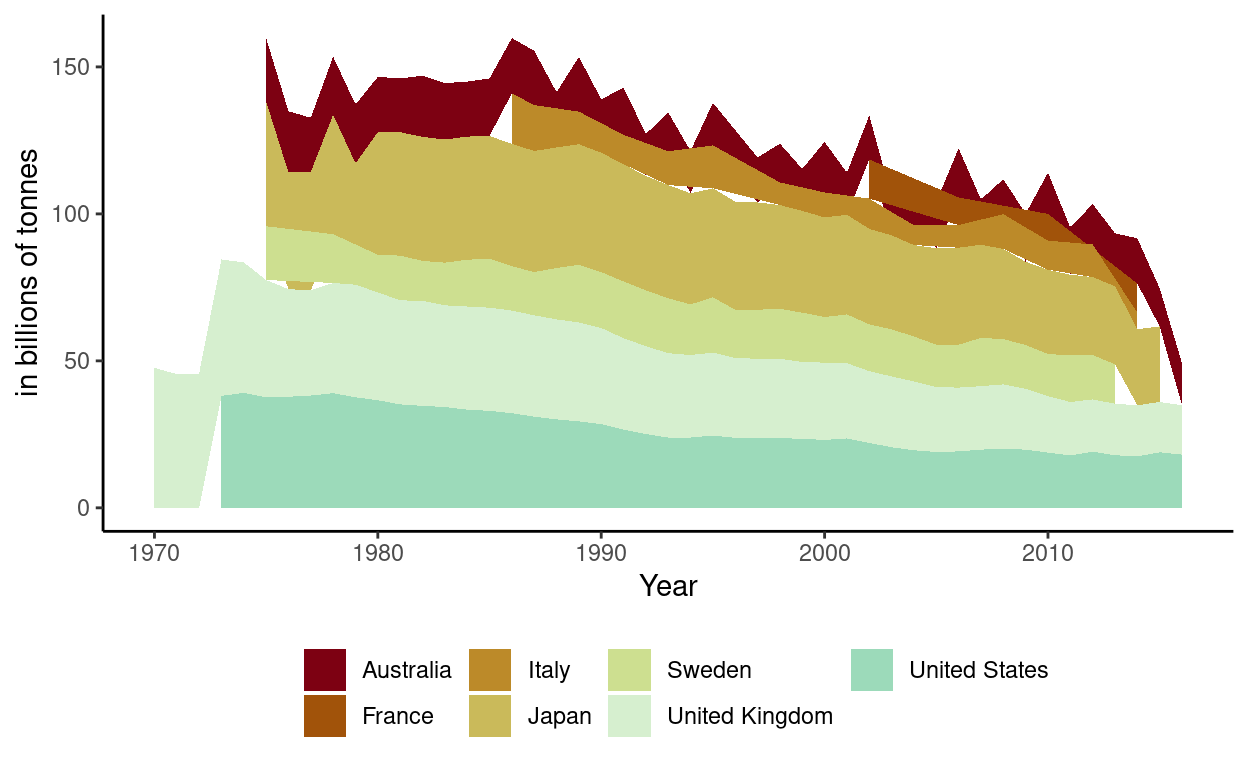# Project Part 2

Interactive and static plots of Wage Gaps from 1970 to 2016

1. Packages I will use to read in and plot the data
``````library(tidyverse)
library(echarts4r)
``````
1. Read the data in from part 1
``````country_wageGap  <- read_csv(here::here("country_wageGap.csv"))
``````

# Interactive graph

• Group_by country so there will be a “river” for each country
• Use e_charts to create an e_charts object with Year on the x axis
• Use e_river to build “rivers” that contain Wage Gaps by country. The depth of each river represents wage gap percentage for each country
• Use e_tooltip to add a tooltip that will display based on the axis values
• Use e_title to add a title, subtitle, and link to subtitle
• Use e_theme to change the theme to roma
``````country_wageGap   %>%
group_by(country)  %>%
e_charts(x = Year)   %>%
e_river(serie = WageGap, legend=FALSE)  %>%
e_tooltip(trigger = "axis")  %>%
e_title(text = "Annual Wage Gap, by country",
subtext = "(in percentages). Source: Our World in Data",
left = "center")  %>%
e_theme("roma")
``````

# Static graph

• Use ggplot to create a new ggplot object. Use aes to indicate that Year will be mapped to the x axis; wage gaps will be mapped to the y axis; Country will be the fill variable
• geom_area will display WageGap
• scale_fill_discrete_divergingx is a function in the colorspace package. It sets the color palette to roma and selects a maximum of 12 colors for the different countries
• theme_classic sets the theme
• theme(legend.position = “bottom”) puts the legend at the bottom of the plot
• labs sets the y axis label, fill = NULL indicates that the fill variable will not have the labelled Country
``````country_wageGap   %>%
ggplot(aes(x = Year, y = WageGap,
fill = country)) +
geom_area() +
colorspace::scale_fill_discrete_divergingx(palette = "roma", nmax =11) +
theme_classic() +
theme(legend.position = "bottom") +
labs( y = "in billions of tonnes",
fill = NULL)
``````These plots show a decrease in wage gaps since 1970. Wage gaps have continued to decrease with occasional spikes.

``````ggsave(filename = here::here("_posts/2022-05-07-project-part-2/preview.png"))
``````